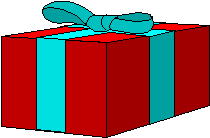#### You may also like### Tweedle Dum and Tweedle Dee

Two brothers were left some money, amounting to an exact number of pounds, to divide between them. DEE undertook the division. "But your heap is larger than mine!" cried DUM...### Sum Equals Product

The sum of the numbers 4 and 1 [1/3] is the same as the product of 4 and 1 [1/3]; that is to say 4 + 1 [1/3] = 4 ï¿½ 1 [1/3]. What other numbers have the sum equal to the product and can this be so for any whole numbers?### Special Sums and Products

Find some examples of pairs of numbers such that their sum is a factor of their product. eg. 4 + 12 = 16 and 4 × 12 = 48 and 16 is a factor of 48.

# Plutarch's Boxes

##### Age 11 to 14Challenge LevelWhat rectangular boxes, with integer sides, have their surface areas equal to their volumes? One example is $4$ by $6$ by $12$. There are $10$ solutions. Can you find them all?

Suppose the dimensions of the box are $a$, $b$ and $c$ units where $a \le b \le c$, then the volume of the box is $abc$ and the surface area is $2(ab+ bc + ca)$. If these are equal to each other you can divide the expression you get by abc to give:

$1 = \frac{2}{a} + \frac{2}{b} + \frac{2}{c}$

Now you have $3$ positive numbers (fractions) adding up to $1$ and there are only a few possible ways this can happen. None of the fractions can be very small or very big. You need to show that

$3 \le a \le 6 , 3 \le b \le 12 , 3 \le c \le 144.$

This limits the number of possibilities. Knowing how far to go in the search, it is then easy to write a short program to find all possible boxes. You could use a spreadsheet. You could just go through all possible cases systematically as people would have done before the days of computers.

According to Plutarch, the Greeks found all the rectangles with integer sides, whose areas are equal to their perimeters. Can you find them? You can use the technique described above in this simpler case.